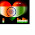### IBPS /SBI PO BANKING STUDY MATERIAL ON QUANTITATIVE APTITUDE TRAINS

TRAINS SHORTCUTS FOR QUANTITATIVE APTITUDE
1. When a train passes a stationary point, the distance covered (in the passing) is the length of the train.
Eg 1: What is the time taken by a train of length 360m to cross a pole at a speed of 72 kmph ?

Ans: Time taken by the train to cross the pole = Length of the train / Speed of the train
Speed of the train is given in kmph, whereas the length of the train is given in mts. So the speed of the train is to be expressed in m/sec.
Speed of the train ( in mts/sec) = 72 x (5/18)
= 20 mts/sec
Time taken by the train to cross the pole = 360/20
= 18 seconds.
2. If the train is crossing a platform or a bridge, the distance covered by the train is equal to the length of the train plus the length of the platform or a bridge.
Eg 2: How long will a train 200 m long travelling at a speed of 54 kmph take to cross a platform of length 100 m?
Ans: Distance covered by the train = Length of the train + Length of the platform
= 200 + 100
= 300 m
Speed of the train is given in kmph, whereas the distance covered by the train is given in mts. So speed of the train is to be expressed in mts / sec.
Speed of the train = 54 x (5/18)
= 15 mts/ sec
Time taken by the train = 300/15
= 20 seconds.
3. If two trains pass each other ( travelling in the same direction or in opposite directions) , the total distance covered ( in the crossing/ overtaking as the case may be) is equal to the sum of the lengths of the two trains.
Eg3: Two trains 121 mts and 99 mts in length respectively are running in opposite directions, one at the rate of 40 kmph and the other at the rate of 32 kmph. In what time will they be completely clear of each other from the moment they meet?
Ans: As the trains are moving in opposite directions their relative speed = 40 + 32 km/hr.
= 72 km/hr.
The length to be travelled by the trains = 121 + 99
= 220 mts.
The speed of the train is given in Kmph, whereas the length is given in mts. Hence, the relative speed of the trains is to be expressed in mts/sec.
The relative speed in mts/sec = 72 x (5/18)
= 20 mts /sec
Time required to completely clear of each other from the moment they meet = 220 / 20
= 11 secs.

4. If two bodies are moving in the same direction at speeds S1 and S2 respectively, then the relative speed is:
Relative speed = S1 - S2
5. If two bodies are moving in opposite direction at speeds S1 and S2 respectively, then the relative speed is:
Relative speed = S1+ S2.
6. Two trains of length 'p' m and 'q' m respectively run on parallel lines of rails. When running in the same direction the faster train passes the slower one in 'a' seconds, but when they are running in opposite directions with the same speeds as earlier, they pass each other in 'b' seconds. Then,
Speed of the faster train = [( p + q)/ 2] x [ ( a+b) / (a x b)]
Speed of the slower train = [(p-q) / 2] x [ (a-b) / (a x b)]
Note : The speeds obtained using the above formula are in mts/ sec, if the speeds are to be expressed in kmph, they have to be multiplied by 18/5.
Eg 4: Two trains of length 100 m and 250 m run on parallel lines. When they run in the same direction it will take 70 seconds to cross each other and when they run in opposite direction, they take 10 seconds to cross each other. Find the speeds of the two trains?
Ans: Speed of the faster train = [(100 + 250) / 2] [ (70 + 10) / ( 70 x 10) ].
= 175 x (8 /70)
= 20 m/sec.
Speed of the slower train = [ ( 100 + 250) / 2] [ ( 70-10) / (70 x 10) ]
= 175 x ( 6/ 70)
= 15 m/ sec.

Therefore, speeds of the trains are 72 kmph (20 x 18 /5) and 54 kmph ( 15 x 18/5) respectively.
6. If a train passes by a stationary man in 'p' seconds and passes by a platform / bridge, the length of which is 'm' mts, completely in 'q' sec. Then
Length of the train = (m x p) / (q-p).
Eg 5: A train crosses by a stationary man standing on the platform in 7 seconds and passes by the platform completely in 28 seconds. If the length of the platform is 330 meters, what is the length of the train?
Ans: Length of the train = ( 330 x 7) / ( 28-7)
= 330x 7 / 21
FOR PURCHASING BANKING MATERIAL PLEASE CALL 09038870684 OR MAIL AT tamal253@yahoo.com

### Comments